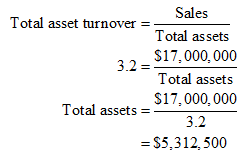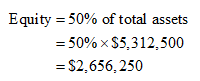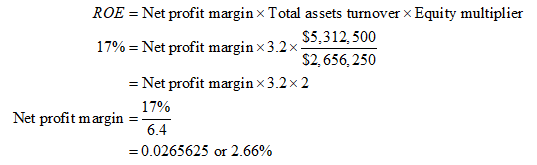# PMM has \$17 million in sales, its ROE is 17%, and its total asset turnover is 3.2X.  Common equity on the firms balance sheet is 50% of its total assets.  What is the net income?

Question
1 views

PMM has \$17 million in sales, its ROE is 17%, and its total asset turnover is 3.2X.  Common equity on the firms balance sheet is 50% of its total assets.  What is the net income?

check_circle

Step 1: Calculate the total assets as follows:Step 2: Calculate the value of equity as follows:Step 3: Calculate the net profit margin as follows:...

### Want to see the full answer?

See Solution

#### Want to see this answer and more?

Solutions are written by subject experts who are available 24/7. Questions are typically answered within 1 hour.*

See Solution
*Response times may vary by subject and question.
Tagged in